Back to "random and silly" pages

## Encounters with Goldbach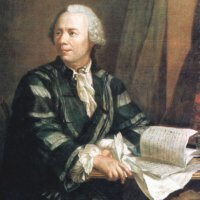The Goldbach conjecture is fascinating for kids already. One can understand the statement in elementary school: the conjecture claims that all even numbers larger than 2 can be written as the sum of two primes. I myself also learned about the conjecture in middle school in 7th grade. My further encounters with the Goldbach conjecture appear to fit well into my "random and silly" online collection, but I also want to see this page also as a contribution to the 300'th birthday of my compatriot Leonard Euler. Eulers exchanged not only many letters with Goldbach, his work has many connections with that conjecture. What follows are some personal encounters with the conjecture. I'm not a number theorist and never worked on that problem. Anyway, the few mathematical formulas on this page should be accessible on a high school or early college level and - except maybe with the exception of the Cauchy formula - by Euler himself. Happy birthday, Leonard! June 2016: Something more about Goldbach in division algebras and for Eisenstein integers. October 23, 2018: Goldbach comets Mathtable Handout 4 pages [PDF] and Goldbach comet Slides 52 Pages [PDF]

### Highschool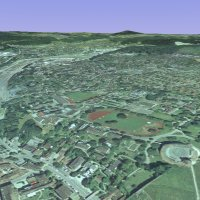In Highschool the "Kantonsschule Schaffhausen", we had a small, but unusual and interesting mathematics library which also featured an Olivetti P 6040 computer. (Here are three programs, I wrote for that machine [PDF'). The small room with the library, a wooden seminar table and computer was only accessible to students who got a key. Since I was one of the two lucky students who got access by our math teacher Roland Stärk, I also had access to that library. Besides books on graph theory and topology - both of which appeared to me as very strange mathematical topics at first -, I tried during a summer to dig into the "Synthetische Zahlentheorie" of Rudolph Fueter [Scan of book cover and table of content] and Vinogradovs book on number theory.[Book cover scan] I found the complexity of the material frustrating. How could a simple result like Vinogradovs theorem that a sufficiently large odd number can be written as the sum of three odd primes, lead to such complex mathematics? I decided to find out and study mathematics in college.

### College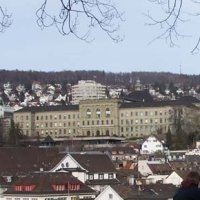At ETH Zürich, I continued to be mesmerized by computers. The work on the early Macintosh computers was rather cumbersome: every student had to carry around large floppies, one with the OS, one with our own stuff. Also the operating system was impossible. I therefore increasingly liked to work on the Math departments UNIX computer, a monstrous VAX computer running Ultrix, a variant of Unix. The Unix operating system fits very well the mathematicians mind, because it is modular, simple and beautiful, like mathematics. Together with a friend Rolf Kannenberg, we once occupied the machine to find counter examples to the Goldbach conjecture. Kannenberg defined the following numbers, which - if he had not called them already Kannenberg numbers himself - I would now call Kannenberg numbers: define a function K(p) on the set P of prime numbers as ```K(p) = min { 2n | 2n-q is not prime for all prime q smaller than p } ``` Of course, if K(p) is smaller than 2p, for some p, we had a counter example to the Goldbach conjecture.

### A Vax computerWe programmed the VAX to find out how fast K(p) should grow and let it run for days. Because we needed arbitrary large integer arithmetic, we used the programming language Cayley, a computer algebra system which was later renamed Magma. The experiments confirmed that the Kannenberg numbers seemed to grow exponentially fast, that the chance of having K(p)>p appeared slimmer and slimmer as longer as the jobs were running. Unfortunately, our latest run got lost: after a few days, we got angry emails from the system administrator: our program had burned 30'000 Swiss Franks of CPU time into their IT budget. Our batch job got killed. This was the end of our Goldbach conjecture adventure. The sequence of numbers 6,12,30,98,220, ... is today known as the Sloane sequence A025018. This attempt also seemed have been an early end of my experimental number theory efforts, because the next entry after day of the Kannenberg entry in the mathematical diary [1 (blue handwriting by Kannenberg)] shows interest in the recursion xn+2 = xn/(1+xn+1), which got me interested dynamical systems, a topic, which allowed experimentation with computers too and which appeared to me less hopeless than a 300 year old unsolved problem in number theory. My collegue Rolf Kannenberg also graduated in mathematics. I met him a last time as a graduate student, when he was one of my competitors in a 10K race organized by ASVZ in Fluntern. I continued to like to read about number theory in college too. A good book-friend has become the book of Hua, which is accessible for undergraduate students. It contains for example a detailed and managable proof of the Goldbach-Schnirelman theorem that there is a bound c for which all numbers n can be written as a sum of c prime numbers. Added July 2016: The machine was a VAX 11/785 with Ultrix-32 V.1.1 (~BSD-4.2). It came in October 1984 to ETH. The machine was called Bernina and was also the ETH Usenet News server in the 80ies. The machine had 8 Meg of RAM and 1.2 Gig of fixed disk space! Costed fully configured 400'000 Dollars. More info about this VAX 11/785.

### The Kannenberg numbers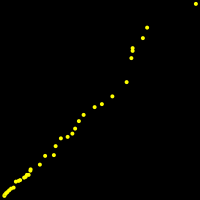Here are the first Kannenberg numbers. The number 98 for example is the first even number for which n-3,n-5,n-7 are all not prime. It is also the smallest even number for which n-3,n-5,n-7,n-11,n-13,n-17 is not prime, but then 220 is the first even number for which n-3,n-5,n-7,n-11,n-13,n-17,n-19 are all not prime: ```6,12,30,98,220,308,556,992,2642,5372,7426,43532,54244,63274, 113672,128168,194428,194470,413572,503222,1077422,3526958, 3807404,10759922,24106882,27789878,37998938,60119912,113632822, 187852862,335070838,419911924,721013438,1847133842,7473202036, 11001080372,12703943222,21248558888,35884080836,105963812462 ``` By the prime number theorem, there are infinitely many Kannenberg numbers because otherwise, prime numbers would have a positive density. The sequence can be generated easily with any computer algebra system. The above numbers (until 1847133842) were obtained with the following Mathematica line: ```p=2; n=3; P=Table[Prime[k],{k,2,1000}]; While[True, k=1; While[Not[PrimeQ[2n-P[[k]]]],k++]; If[P[[k]]>p,Print[{p,2n}]; p=P[[k]]]; n++; ]; ``` on a standard PC in 2 days. The Pari version ```p=2;n=3; { while(1,k=1; while(1-isprime(2*n-prime(k)),k++); if((prime(k)>p),print(2*n);p=prime(k));n++;) } ``` computes things in about the same time but needs less resources (I kept one process running on an unused machine, 7473202036,11001080372,12703943222 needed a few days, 21248558888 an other week and 35884080836 an other two weeks, the last 105963812462 which belongs to the prime 2803 needed several months (P.S. adding the lines parisize = 200M and primelimit = 1000M in the Pari initialization file .gprc. slows startup time of pari but makes things faster.) In Herkomers page the Kannenberg numbers had been computed until 721013438. The picture shows the graph of all numbers (p,[log(K(p)]3).

### Relations with Fourier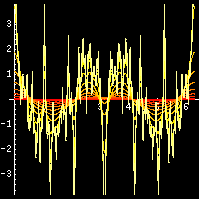My stay at Caltech had been an exciting time: Barry Simon had just launched an ambitious and extensive program of investigating singular continuous spectral properties of Schroedinger operators. I had become interested in Schroedinger operators as a graduate student because they appear as "Jacobeans" in twist dynamical systems. The theory also applies to Koopman operators in ergodic theory. In any case, spectral properties are closely related to dynamical properties of the systems. I'm still happy that I could witness and contribute a tiny bit to this developments. Before leaving from California to the university of Arizona, I briefly became interested in the "prime measure", the pseudo measure m which has the Fourier transform c(n) = 1P(n), where P is the set of prime numbers. This is a rather singular object because for an absolutely continuous measure, the Fourier transform decays and for a discrete measure, the Fourier transform is almost periodic. Is it accessible by Fourier theory? My diary shows that I worried then, how much of the theory for Hausdorff measures carries over to pseudo measures. ```PrimeMeasure[m_]:=Module[{}, P=Table[Prime[k],{k,1,m}]; f[x_]:=Sum[2 Cos[P[[i]] x],{i,2,m}]; Plot[f[x],{x,0,2Pi},AspectRatio->1]]; ``` The properties of the prime numbers are so encoded as generalized function on the circle. What are the properties of this object? What is its support on the circle? What are the properties of the square m2 which corresponds to the convolution of its Fourier transform? If the Goldbach conjecture is true, then all the even Fourier coefficients c2n = < e2n i x, m2 > of m2 are positive. The Fourier approach is nothing else than exponential sum m(x) = sump ei x p and the key to many results in that field.

### Power with generating functions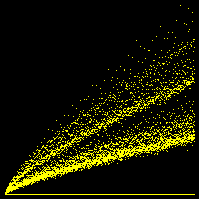Powerful tools to transform a problem into a different problem form the backbone of mathematics. Examples are

 Generating functions Combinatorics sumn p(n) xn = prodi (1-xi) Characteristic functions Probability theory sin(at)/(at) for uniform ddistribution Laplace transform Differential equations f'=f, s F(s) = F(s) + f(0) Fourier transform Partial differential equations ut = ux, U'(k) = k U(k) Dirichlet series Number theory 1/zeta(s) = sumn mu(n)/n^s
The function
```f(z) = sump prime zp = z2 + z3 + z5 + ...
```
is the generating function of the prime numbers. Its square f(z)2 = sumn rn zn generate the numbers r(n) which give the number of times that n can be written as a sum of two primes. This allows to compute r(n) in an elegant way:
```M=10000;h[z_]=Sum[z^Prime[k],{k,M}];ListPlot[CoefficientList[Series[h[z]^2,{z,0,M}],z]]
```
The picture to the left shows the corresponding plot of the Taylor coefficients of
```f(z)2 = z4 + 2 z5 + z6 + 2 z7 +....
```

### A magic bullet?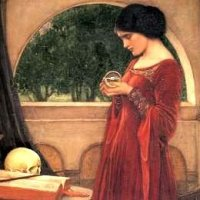Assume we could find a sequence an such that ``` f(x) = sump prime ap ei p x ``` is a function which is explicitly known. Then ``` f(x)2 = sumn bn ei n x ``` and the Goldbach conjecture would mean that ``` b2n = < e2n i x, f2 > ``` is positive for all n. If f were explicitely known, then f2 would be known and the Fourier coefficients could be computed. This might appear naive, because there is no reason, why such a function f should exist. Hardy-Littlewood could squeze out a lot with ap = log(p).

### Eulers golden key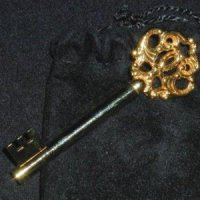An example: if a(n) = -log(1-1/ns), then with z=r ei p x ``` f(z) = sump ap zp = - sump log(1-1/ps) zp ``` which for z=1 by Eulers "golden key" formula equal to ```f(1) = log prodp 1/(1-1/ps) = log (zeta(s) ) ``` The choice of an has at least one computable value.

### Hardy-Littlewoods circle method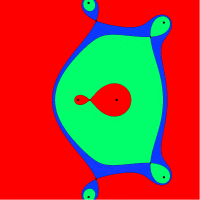Finding a sequence an, for which the Fourier coefficients of f2 can be estimated, is a trick called the "circle method" discovered by Hardy and Littlewood. For bounded sequences an like an, the function ``` f(z) = sump ap zp = a2 p2 + a3 p 3 + ... ``` is analytic in the unit disc. If an decays faster, the function is analytic in the entire plane. Here is a picture of the function ``` f(z) = sump prime zp/p! ``` The image above shows the complex plane colored according to the values of |f(z)|. The name circle method comes from the fact that one is interested in the function on circles.

### Cauchy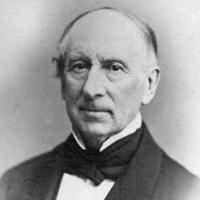The Goldbach conjecture states that all even derivatives of the function f2(z) are nonzero at the origin. Assume, there was a function for which the roots and an explicit formula ```f(z) = prod (z-zi) exp(g(z)) ``` where known, then there could be hope to compute the derivatives of g(z) = f2(z) at 0, possibly with the Cauchy integral formula ```g(n)(0) = n!/(2 pi i) int|z|=r g(z)/z(n+1) dz. ```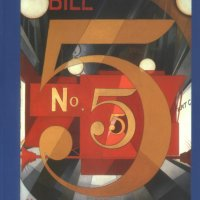In 2004, a multi-variable student of mine Jose Ramirez told me about the book "Uncle Pedros and the Goldbach conjecture" by Apostolos Doxiadis. I subsequently read that book with great joy. I especially liked the part, where the proof attempt was stopped when Uncle Pedros heared about Goedels incompleteness theorem, which would leave the possibility that Goldbachs conjecture is true but not provable. The book has a nice ending which I don't want to give away. Uncle Pedros approach to the conjecture with "beeds" seems a highly unlikely approach. It is more likely that some hard analysis analoge to Vinogradov or a new theory will break the conjecture.

### Of course, it is true !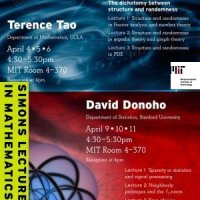In his first Simons lectures at MIT in April 2007 for a general audience, which I watched, Terence Tao was asked, whether he believes that the Goldbach conjecture is true: Tao: "Did you ask, whether I think a proof will be found or whether it is true?" Audience: "Whether it is true". Tao: "Of course it is true! The numerical evidence is overwhelming." Tau's talk mentioned the Goldbach conjecture together with the twin prime conjecture and the Germaine conjecture in the context of a general Dickson conjecture. Tao's talks were structured around the dichotomy "order-random", which in spectral theory ranges from discrete spectrum and absolutely continuous spectrum in dynamical system theory from integrability to hyperbolicity, or in number theory between arithmetic sets and random sets. In all these cases, the interesting structures are between these two extremes or mixtures of them. The prime numbers for example are defined as the complement of a union of arithmetic sets and behave like a random set. It is the pseudo random nature, which makes it challenging.

### Goldbach in the Movies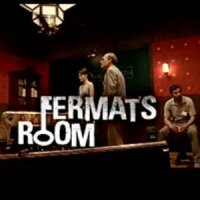Added in July 2009: one of the wonderful things of collecting things online is that readers can give input. In July 2009, Evan Pellnitz suggested to me by email the movie "Fermat's room". I could only order the Spanish version (other region code) but it was worth the trouble. The thriller is fantastic evenso the math riddles are quite standard and well known. You find the start of the movie here and the Teaser on youtube. A young mathematician who claims to have a proof of Goldbach's conjecture is broken in. All his files and notes have disappeared. Shortly after, he is invited with 3 other mathematicians to a mysterious house, where they are entrapped in a room in which the walls are moving in on them. They have to solve math riddles to slow the progress of their execution. Who wants to kill them and why? The solution is linked to Goldbach's conjecture.